UPSC  >  Test: Dice- 2

# Test: Dice- 2

Test Description

## 10 Questions MCQ Test Logical Reasoning (LR) and Data Interpretation (DI) | Test: Dice- 2

Test: Dice- 2 for UPSC 2022 is part of Logical Reasoning (LR) and Data Interpretation (DI) preparation. The Test: Dice- 2 questions and answers have been prepared according to the UPSC exam syllabus.The Test: Dice- 2 MCQs are made for UPSC 2022 Exam. Find important definitions, questions, notes, meanings, examples, exercises, MCQs and online tests for Test: Dice- 2 below.
Solutions of Test: Dice- 2 questions in English are available as part of our Logical Reasoning (LR) and Data Interpretation (DI) for UPSC & Test: Dice- 2 solutions in Hindi for Logical Reasoning (LR) and Data Interpretation (DI) course. Download more important topics, notes, lectures and mock test series for UPSC Exam by signing up for free. Attempt Test: Dice- 2 | 10 questions in 10 minutes | Mock test for UPSC preparation | Free important questions MCQ to study Logical Reasoning (LR) and Data Interpretation (DI) for UPSC Exam | Download free PDF with solutions
 1 Crore+ students have signed up on EduRev. Have you?
Test: Dice- 2 - Question 1

### Directions to Solve Six dice with upper faces erased are as shows.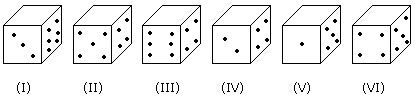The sum of the numbers of dots on the opposite face is 7. Question - If the odd numbered dice have even number of dots on their top faces, then what would be the total number of dots on the top faces of their dice?

Detailed Solution for Test: Dice- 2 - Question 1

Odd numbered dice are : (I), (III) and (V)

No. of dots on the top faces of these dice are 2, 2 and 4 respectively.

Required total = 2 + 2 + 4 = 8

Test: Dice- 2 - Question 2

### Directions to Solve Six dice with upper faces erased are as shows.The sum of the numbers of dots on the opposite face is 7. Question - If even numbered dice have even number of dots on their top faces, then what would be the total number of dots on the top faces of their dice?

Detailed Solution for Test: Dice- 2 - Question 2

Even numbered dice are: (II), (IV) and (VI)

No. of dots on the top face of (II) dice = 6

No. of dots on the top face of (IV) dice = 6

and No. of dots on the top face of (VI) dice = 6

Therefore Required total = 6 + 6 + 6 = 18

Test: Dice- 2 - Question 3

### Directions to Solve Six dice with upper faces erased are as shows.The sum of the numbers of dots on the opposite face is 7. Question - If dice (I), (II) and (III) have even number of dots on their bottom faces and the dice (IV), (V) and (VI) have odd number of dots on their top faces, then what would be the difference in the total number of top faces between there two sets?

Detailed Solution for Test: Dice- 2 - Question 3

No. of faces on the top faces of the dice (I), (II) and (III) are 5, 1 and 5 respectively.

Therefore, Total of these numbers = 5 + 1 + 5 = 11

No. of dots on the top faces of the dice (IV), (V) and (VI) are 1, 3 and 1 respectively.

Therefore, Total of these numbers = 1 + 3 + 1 = 5

Required difference = 11 - 5 = 6

Test: Dice- 2 - Question 4

Directions to Solve

Six dice with upper faces erased are as shows.The sum of the numbers of dots on the opposite face is 7.

Question -

If the even numbers of dice have odd number of dots on their top faces and odd numbered dice have even of dots on their bottom faces, then what would be the total number of dots on their top faces?

Detailed Solution for Test: Dice- 2 - Question 4

No. of dots on the top faces of the dice (II), (IV) and (VI) are 1, 1 and 1 respectively.

No. of dots on the top faces of the dice (I), (III) and (V) are 5, 5 and 3 respectively.

Required total = 5 + 5 + 3 + 1 + 1 + 1 = 16

Test: Dice- 2 - Question 5

Directions to Solve

Six dice with upper faces erased are as shows.The sum of the numbers of dots on the opposite face is 7.

Question -

If the dice (I), (II) and (III) have even number of dots on their bottom faces, then what would be the total number of dots on their top faces?

Detailed Solution for Test: Dice- 2 - Question 5

No. of dots on the top faces of dice (I), (II) and (III) are 5, 1 and 5 respectively.

Required total = 5 + 1 + 5 = 11

Test: Dice- 2 - Question 6

Directions to Solve

The figure given on the left hand side in each of the following questions is folded to form a box. Choose from the alternatives (1), (2), (3) and (4) the boxes that is similar to the box formed.

Question -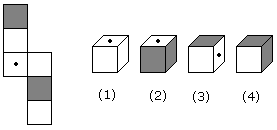Detailed Solution for Test: Dice- 2 - Question 6

As, shown in figure, these are opposite of each other. Thus, option (2) is correct.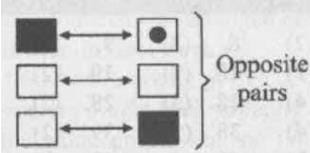Test: Dice- 2 - Question 7

Directions to Solve

The figure given on the left hand side in each of the following questions is folded to form a box. Choose from the alternatives (1), (2), (3) and (4) the boxes that is similar to the box formed.

Question -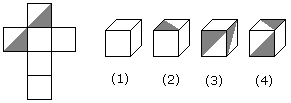Test: Dice- 2 - Question 8

Directions to Solve

The figure given on the left hand side in each of the following questions is folded to form a box. Choose from the alternatives (1), (2), (3) and (4) the boxes that is similar to the box formed.

Question -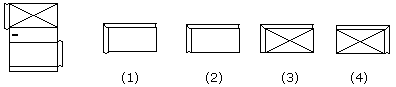Detailed Solution for Test: Dice- 2 - Question 8

The pattern on fig. (X) and also the fact that the faces are rectangle, indicate that only fig. (1) can be obtained by folding fig. (X).

Test: Dice- 2 - Question 9

Directions to Solve

The figure given on the left hand side in each of the following questions is folded to form a box. Choose from the alternatives (1), (2), (3) and (4) the boxes that is similar to the box formed.

Question -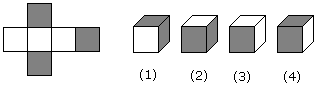Test: Dice- 2 - Question 10

Directions to Solve

The figure given on the left hand side in each of the following questions is folded to form a box. Choose from the alternatives (1), (2), (3) and (4) the boxes that is similar to the box formed.

Question -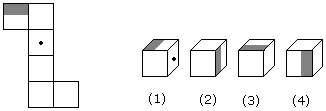## Logical Reasoning (LR) and Data Interpretation (DI)

96 videos|83 docs|119 tests
 Use Code STAYHOME200 and get INR 200 additional OFF Use Coupon Code
Information about Test: Dice- 2 Page
In this test you can find the Exam questions for Test: Dice- 2 solved & explained in the simplest way possible. Besides giving Questions and answers for Test: Dice- 2, EduRev gives you an ample number of Online tests for practice

## Logical Reasoning (LR) and Data Interpretation (DI)

96 videos|83 docs|119 tests

### How to Prepare for UPSC

Read our guide to prepare for UPSC which is created by Toppers & the best Teachers